# Diophantus

Diophantus of Alexandria (Greek: Διόφαντος ὁ Ἀλεξανδρεύς) (c. 214 - c. 298 C.E.) was a Hellenistic mathematician. He is sometimes called "the Father of Algebra," a title he shares with Muhammad ibn Musa al-Khwarizmi. He is the author of a series of classical mathematical books called, The Arithmetica, and worked with equations which are now called Diophantine equations; the method to solve those problems is now called Diophantine analysis. The study of Diophantine equations is one of the central areas of number theory. Diophantus also authored a tract "On Polygonal Numbers" and a collection of propositions called Porisms. The findings and works of Diophantus have influenced mathematics greatly and caused many other questions to arise. The most famous of these is Fermat's Last Theorem.

Diophantus also made advances in mathematical notation and was the first Hellenistic mathematician who frankly recognized fractions as numbers.

## Biography

Little is known about the life of Diophantus. He lived in Alexandria, Egypt, probably from between 200 and 214 to 284 or 298 C.E. There is still a lot of speculation as to when he lived. According to Rafael Bombelli's Algebra, published in 1572, Diophantus lived under Antoninus Pius (138-161 C.E.), but there is no proof. Another source, a letter of Psellus (eleventh century), mentions Diophantus and Anatolius as writers on the Egyptian method of reckoning. It is taken, from that source, that Diophantus most probably flourished around 250 C.E. Most scholars consider Diophantus to have been a Greek, though it has been suggested that he may have been a Hellenized Babylonian.

Almost everything known about Diophantus comes from a single fifth century Greek anthology, which is a collection of number games and strategy puzzles. One of the puzzles is:

This tomb holds Diophantus. Ah, what a marvel! And the tomb tells scientifically the measure of his life. God vouchsafed that he should be a boy for the sixth part of his life; when a twelfth was added, his cheeks acquired a beard; He kindled for him the light of marriage after a seventh, and in the fifth year after his marriage He granted him a son. Alas! late-begotten and miserable child, when he had reached the measure of half his father's life, the chill grave took him. After consoling his grief by this science of numbers for four years, he reached the end of his life.

The translation and solution of this epigram-problem infers that Diophantus' boyhood lasted fourteen years, acquired a beard at 21, and married at age 33. He fathered a son five years later, but that son died at age 42—Diophantus, at this time, was 80 years old. He tried to distract himself from the grief with the science of numbers, and died 4 years later, at 84.

This puzzle reveals that Diophantus lived to be about 84 years old. It is not certain if this puzzle is accurate or not.

## Arithmetica

The Arithmetica is the major work of Diophantus and the most prominent work on algebra in Greek mathematics. It is a collection of problems giving numerical solutions of both determinate and indeterminate equations. Of the original thirteen books of which Arithmetica consisted, only six have survived, though there are some who believe that four Arab books discovered in 1968 are also by Diophantus. Some Diophantine problems from Arithmetica have been found in Arabic sources.

### History

After Diophantus's death, the Dark Ages began, spreading a shadow on math and science, and causing knowledge of Diophantus and the Arithmetica to be lost in Europe for roughly 1500 years. Sir Heath stated in his Diophantus of Alexandria, "After the loss of Egypt, the work of Diophantus long remained almost unknown among the Byzantines; perhaps one copy only survived (of the Hypatian recension), which was seen by Michael Psellus and possibly by the scholiast to Iamblichus, but of which no trace can be found after the capture of Constantinople in 1204." Possibly the only reason that some of his work has survived is that many Arab scholars studied his works and preserved this knowledge for later generations. In 1463, German mathematician Regiomontanus wrote: “No one has yet translated from the Greek into Latin the thirteen Books of Diophantus, in which the very flower of the whole of arithmetic lies hidden….”

The first Latin translation of Arithmetica was by Bombelli who translated much of the work in 1570, but it was never published. Bombelli did, however, borrow many of Diophantus's problems for his own book, Algebra. The editio princeps of Arithmetica was published in 1575, by Xylander. The most famous Latin translation of Arithmetica was by Bachet in 1621, which was the first translation of Arithmetica available to the public.

### Margin writing by Fermat and Planudes

The 1621 edition of Arithmetica by Bombelli gained fame after Pierre de Fermat wrote his famous "Last Theorem" in the margins of his copy:

“If an integer n is greater than 2, then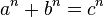$a^n + b^n = c^n$ has no solutions in non-zero integers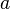$a$,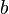$b$, and$c$. I have a truly marvelous proof of this proposition which this margin is too narrow to contain.”

Fermat's proof was never found, and the problem of finding a proof for the theorem went unsolved for centuries. A proof was finally found in 1994, by Andrew Wiles after working on it for seven years. It is believed that Fermat did not actually have the proof he claimed to have. Although the original copy in which Fermat wrote this is lost today, Fermat's son edited the next edition of Diophantus, published in 1670. Even though the text is otherwise inferior to the 1621 edition, Fermat's annotations—including his famous "Last Theorem"—were printed in this version.

Fermat was not the first mathematician so moved to write in his own marginal notes to Diophantus; the Byzantine mathematician Maximus Planudes had written "Thy soul, Diophantus, be with Satan because of the difficulty of your theorems" next to the same problem.

## Other works

Diophantus did not just write Arithmetica, but very few of his other works have survived. He authored a tract, "On Polygonal Numbers," and a collection of propositions, called Porisms.

### The Porisms

Diophantus himself refers to a work which consists of a collection of lemmas called The Porisms (or Porismata), but this book is entirely lost. Many scholars and researchers believe that The Porisms may have actually been a section included inside Arithmetica or it may have been the rest of Arithmetica. Although The Porisms is lost, three lemmas contained in The Porisms are known because Diophantus refers to them in Arithmetica.

One such lemma is that the difference of the cubes of two rational numbers is equal to the sum of the cubes of two other rational numbers; that is, given any numbers$a$,$b$ then there exist numbers$c$ and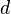$d$ such that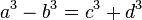$a^3 -b^3= c^3 + d^3$.

### On polygonal numbers and geometric elements

Diophantus is also known to have written on polygonal numbers. Fragments of one of Diophantus' books on polygonal numbers, a topic of great interest to Pythagoras and his followers, has survived. An extant work called Preliminaries to the Geometric Elements, which has been attributed to Hero of Alexandria, has been studied recently and it is suggested that the attribution to Hero is incorrect, and that the work is actually by Diophantus.

## Influence

Diophantus' work has had a large influence in history. Editions of Arithmetica exerted a profound influence on the development of algebra in Europe in the late sixteenth and through the seventeenth and eighteenth centuries. Diophantus and his works have also influenced Arab mathematics and were of great fame among Arab mathematicians. Diophantus' work created a foundation for work on algebra and, in fact, much of advanced mathematics is based on algebra. As far as is known, Diophantus did not affect the lands of the Orient much and how much he affected India is a matter of debate.

### The father of Algebra?

Diophantus is often called “the Father of Algebra" because he contributed greatly to number theory, mathematical notation, and because Arithmetica contains the earliest known use of syncopated notation. However, it seems that many of the methods for solving linear and quadratic equations used by Diophantus go back to Babylonian mathematics. For this reason, mathematical historian Kurt Vogel writes: “Diophantus was not, as he has often been called, the Father of Algebra. Nevertheless, his remarkable, if unsystematic, collection of indeterminate problems is a singular achievement that was not fully appreciated and further developed until much later.”

According to some historians of mathematics, like Florian Cajori, Diophantus got the first knowledge of algebra from India, although other historians disagree.

## Diophantine analysis

Today, Diophantine analysis is the area of study where integral (whole number) solutions are sought for equations, and Diophantine equations are polynomial equations with integral coefficients to which only integral solutions are sought. It is usually rather difficult to tell whether a given Diophantine equation is solvable. Most of the problems in Arithmetica lead to quadratic equations. Diophantus looked at 3 different types of quadratic equations:$ax^2 + bx = c$,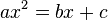$ax^2 = bx + c$, and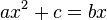$ax^2 + c = bx$.

The reason why there were three cases to Diophantus, while today there is only one, is that he did not have any notion for zero and he avoided negative coefficients by considering the given numbers$a, b, c$ to all be positive in each of the three cases above. Diophantus was always satisfied with a rational solution and did not require a whole number, which means he accepted fractions as solutions to his problems.

Diophantus considered negative or irrational square root solutions "useless," "meaningless," and even "absurd." To give one specific example, he calls the equation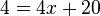$4 = 4x + 20$ 'absurd' because it would lead to a negative value for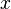$x$. One solution was all he looked for in a quadratic equation. There is no evidence that suggests Diophantus even realized that there could be two solutions to a quadratic equation. He also considered simultaneous quadratic equations.

There are no general comprehensive methods of solving used by Diophantus (that is found). Heath stated, "every question requires a quite special method, which often will not serve even for the most closely allied problems. It is on that account difficult for a modern mathematician even after studying 100 Diophantine solutions to solve the 101st problem; and if we have made the attempt, and after some vein endeavors read Diophantus' own solution, we shall be astonished to see how suddenly he leaves the broad high-road, dashes into a side-path and with a quich turn reaches the goal, often enough a goal with reaching which we should not be content; we expected to have to climb a toilsome path, but to be rewarded at the end by an extensive view; instead of which out guide leads by narrow, strange, but smooth ways to a small eminence; he has finished!"

## Mathematical notation

Diophantus made important advances in mathematical notation. He was the first person to use algebraic notation and symbolism. Before him, everyone wrote out equations completely. Diophantus introduced an algebraic symbolism that used an abridged notation for frequently occurring operations, and an abbreviation for the unknown and for the powers of the unknown. Mathematical historian Kurt Vogel states:

“The symbolism that Diophantus introduced for the first time, and undoubtedly devised himself, provided a short and readily comprehensible means of expressing an equation… Since an abbreviation is also employed for the word ‘equals,' Diophantus took a fundamental step from verbal algebra towards symbolic algebra.”

Although Diophantus made important advances in symbolism, he still lacked the necessary notation to express more general methods. This caused his work to be more concerned with particular problems rather than general situations. For example, Diophantus lacked symbols for the operation of multiplication; this probably became as such since his coefficients are all definite numbers or fractions, and the results are recorded without showing previous work leading to the result. Some of the limitations of Diophantus' notation are that he only had notation for one unknown and, when problems involved more than a single unknown, Diophantus was reduced to expressing "first unknown," "second unknown," etc. in words. He also lacked a symbol for a general number n. Where one would write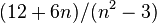$(12 + 6n)/(n^2 -3)$, Diophantus has to resort to constructions like : … a sixfold number increased by twelve, which is divided by the difference by which the square of the number exceeds three.

Heath stated, "Diophantus evidently put down his equations in the ordinary course of writing, i.e. they were written straight on, as are the steps in the propositions of Euclid, and not put in separate lines for each step in the process of simplification."

Algebra still had a long way to go before very general problems could be written down and solved succinctly.